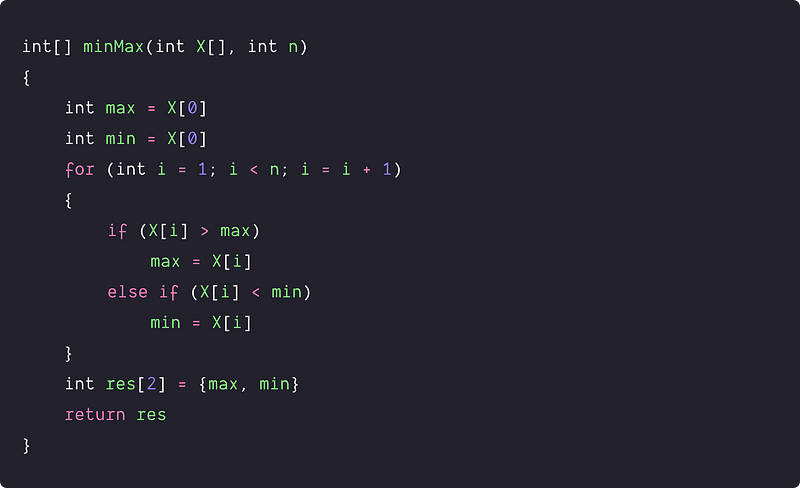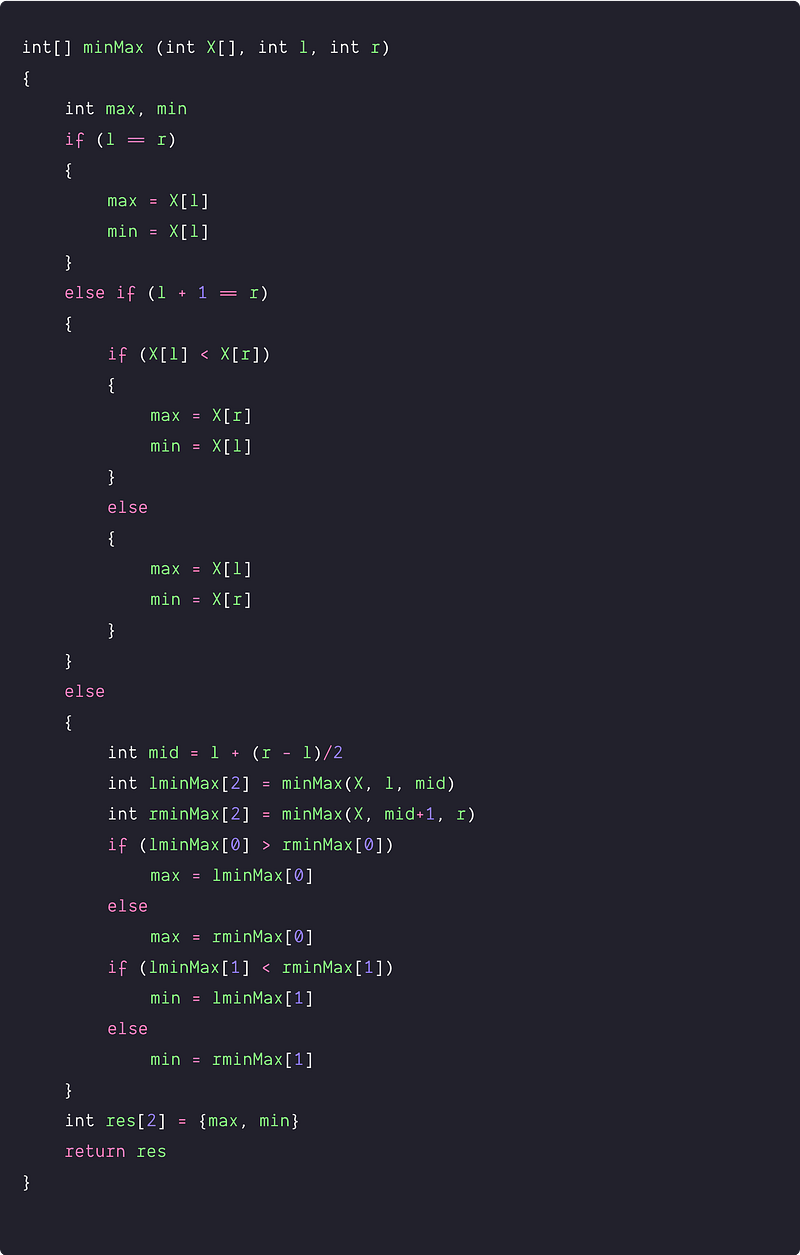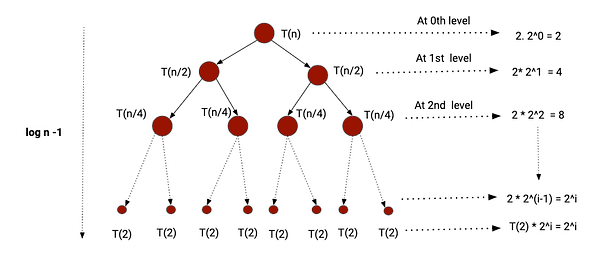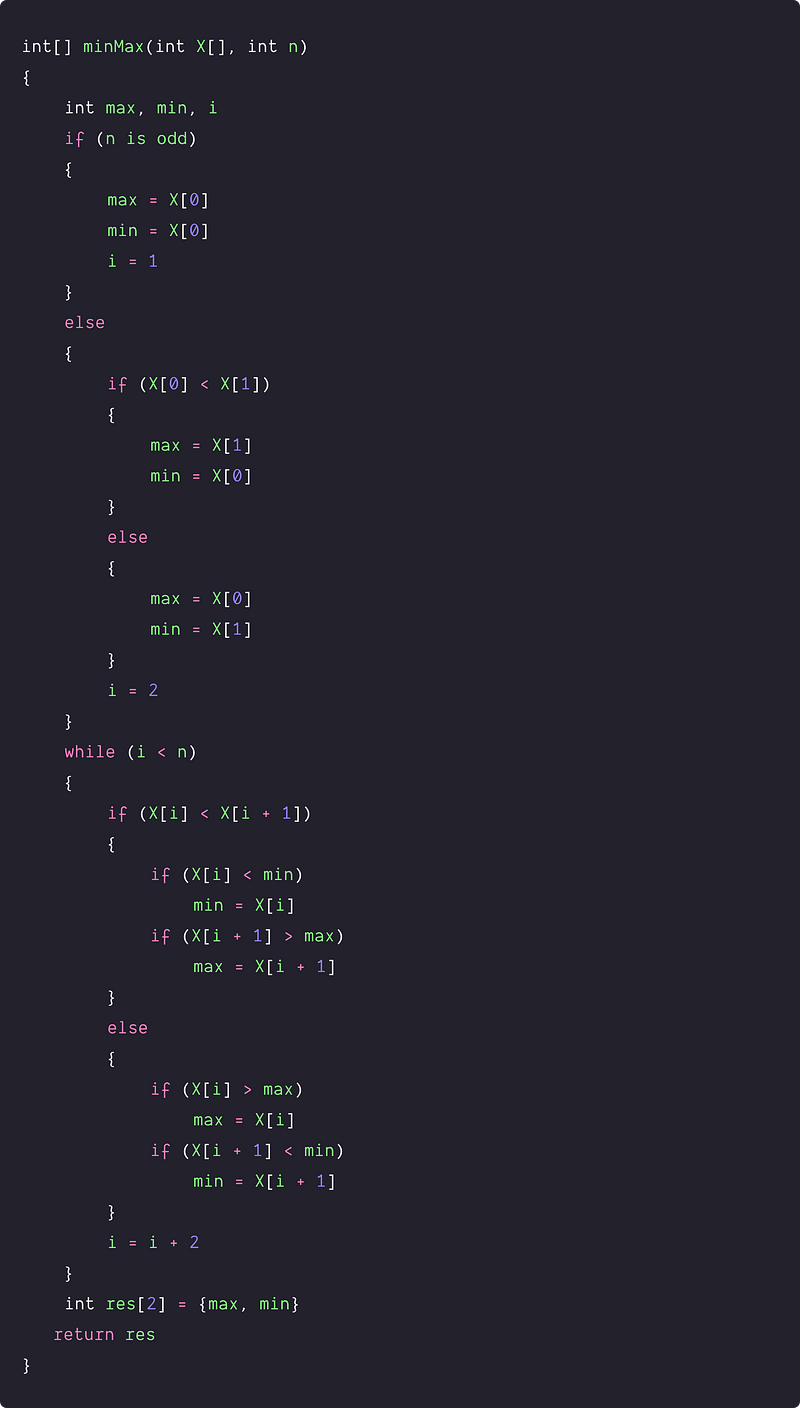# Find the minimum and maximum value in an array

Key takeaways

• A good coding problem to learn problem-solving using single scan and divide and conquer approach.
• We are incrementing the loop by two (or constant) to optimize the code further. We can use a similar idea to optimize code for other coding problems.
• Base conditions, initializations, and if statements are intuitive. We can find similar patterns in other coding problems.
• The time complexities of all three approaches are O(n), but the total comparison count is different. Such a scenario always arises in the solution of other coding problems where we can reduce the count of critical operations to optimize the solution further.

### Let’s understand the problem

Given an array X[] of size n, we need to find the maximum and minimum element present in the array. Our algorithm should make the minimum number of comparisons.

Examples

Input: X[] = [4, 2, 0, 8, 20, 9, 2], Output: max = 20, min = 0

Input: X[] = [-8, -3, -10, -32, -1], Output: max =  -1, min = -32

Important Note: Before moving to the solutions, we recommend learners solve this problem. If solved, then well done! We would like to hear your ideas in the comment. Otherwise no problem, this is an opportunity to learn a new pattern in problem-solving!

Follow-up questions for the interviewer

• Candidate: Are the input values unique? Interviewer: Yes, for the convenience of the solution, we can assume that.
• Candidate: Do we need to solve this problem in-place? Interviewer: Yes, we are looking for an O(n) in-place solution with the minimum number of comparison counts.

Now we are moving forward and discussing the solution ideas in a step-by-step manner. Practicing these steps could help us to arrive at an efficient solution during a coding interview.

### Discussed solution approaches

1. A brute force approach using single scan: loop increment by 1
2. Using divide and conquer: Idea similar to merge sort
3. An efficient approach using single scan: loop increment by 2

### A brute force using single scan: loop increment by 1

Solution idea and steps

• We declare and initialize variables max and min to store maximum and minimum.
• Traverse the array from i = 1 to n-1 and compare each element with min and max.
• If (X[i] < min), it means we have found a value smaller than minimum value till ith index. We update min with X[i] i.e min = X[i].
• else if(X[i] > max), it means we have found a value greater than maximum value till ith index. We update max with X[i] i.e max = X[i].
• By the end of the loop, the minimum and maximum values of the array will be stored in the variables min and max. To return this, we take extra array res[] of size two, where we store maximum on the first index and minimum on the second index. We return the res array as an output.

Solution PseudocodeTime and space complexity analysis

• In the worst case, we are making two comparisons at each step of the iteration. This case will arise if the array is sorted in descending order. In this situation, the first if statement will be false every time, and the second if the statement is true every time. Total no. of comparisons in the worst case = 2*(n-1) = 2n - 2
• The best-case occurs when elements are sorted in ascending order. In this situation, a total of n-1 comparisons have been made. (Think!)

Time complexity = O(n), Space complexity = O(1)

### Using divide and conquer: Idea similar to the merge sort

Solution Idea

Now the critical question is: can we solve the problem using another approach? can we think recursively to arrive at an efficient solution? Here is an idea!

• Divide: divide the array into two equal parts around mid-value i.e divide the problem into two equal-size sub-problems.
• Conquer: Recursively find the minimum and maximum of both left and right parts.
• Combine: Now we compare the maximum and minimum of both halves to get the maximum and minimum of the whole array.

The above idea looks similar to the divide and conquers idea of merge sort. Think!

Solution Steps

• Write a recursive function accepting the array and its start and end index as parameters i.e minMax (int X[], int l, int r)
• Base case 1: If array size is 1, return the element as both max and min.
• Base case 2: If the array size is 2, compare the two elements and return maximum and minimum.

``````if (l == r)
{
max = X[l]
min = X[l]
}
else if (l + 1 == r)
{
if (X[l] < X[r])
{
max = X[r]
min = X[l]
}
else
{
max = X[l]
min = X[r]
}
}
``````
• Divide part: divide the array into two equal parts i.e. mid = l + (r — l)/2
• Conquer part
• Recursively calculate and store the maximum and minimum for the left part i.e. lminMax = minMax(X, l, mid)
• Recursively calculate and store the maximum and minimum for the right part i.e. rminMax = minMax(X, mid+1, r)
• Combine part: Now calculate the overall maximum and minimum by comparing the min and max of both halves. We need to do two comparisons only.

``````if (lminMax > rminMax)
max = lminMax
else
max = rminMax
if (lminMax < rminMax)
min = lminMax
else
min = rminMax
``````
• Store max and min in an extra memory res return it.

Solution PseudocodeTime and space complexity analysis

Let’s define the recurrence relation to analyze the time complexity. Let's T(n) is the time complexity of the problem of input size n and we are dividing the problem into two equal-size sub-problems of size n/2.

T(n) = T(n/2) + T(n/2) + 2 = 2 T(n/2) + 2, where T(2) = 1 and T(1) = 0

We can solve this recurrence relation accurately using recursion tree method. For a better analysis, let’s assume n is a power of 2.Recursion will stop when, n/2^i = 2 => n = 2^(i+1) ……. (1)

In the above recursion tree, the total count of comparison operations
= Sum of comparison count at each level
= 2 + 4 + 8 + 2^i + 2^i
= 2 (2^i — 1) + 2^i (From the sum of the geometric series)
= 2^(i+1) + 2^i — 2
= n + n/2–2 = 3n/2–2

if n is not a power of 2, it does more than 3n/2 -2 comparisons.
=> Time complexity = O(n)
=> Space complexity = Height of the recursion tree = O(logn), for recursion call stack.
Note — Here time complexity is also O(n) but the total number of the comparison operation is less than the previous approach.

### An efficient approach  using single scan: loop Increment by 2

Solution Idea

In the first approach, we are doing two comparison operations for every element in the worst-case scenario. Now the critical question : can we optimize it further and reduce the total number of comparison operations? Let’s think!!

One idea is — let's pick the elements in pairs and try to update the minimum and maximum. Suppose till (i-1)th index, maximum and minimum have been updated in the max and min variable. Now we are considering a pair of ith and (i+1)th index in the next iteration.

• Scenario 1 :  if (X[i] < X[i+1]), then there can be a chance that X[i] can be less than the minimum and X[i+1] can be greater than maximum.

if (X[i] < min) => min = X[i]

if(X[i+1] > max) => max = X[i+1]

• Scenario 2 : if (X[i] > X[i+1]), then there can be a chance that X[i] can be greater than maximum and X[i+1] can be less than the minimum.

if (X[i] > max) => max = X[i]

if (X[i+1] < min) => min = X[i+1]

In both the scenario, we are only doing 3 comparisons (worst case) to update the maximum and minimum of 2 elements. I think we are saving one comparison with respect to the first approach where we need 4 comparisons for 2 elements (worst case).

Initialization : If the array size is odd, we initialize the first element as both min and max, and if it’s even, we compare the first two elements and initialize min and max accordingly.

Solution Steps

• Declare the max and min variables. Check for the size of the array
• If odd, initialize min and max to the first element
• If even, compare the elements and set min to the smaller value and max to the bigger value

``````if (n is odd)
{
max = X
min = X
i = 1
}
else
{
if (X < X)
{
max = X
min = X
}
else
{
max = X
min = X
}
i = 2
}
``````
• Now traverse the array and pick elements in pairs. For each pair (i, i+1), compare both the elements. On the basis of comparison:
• Compare the larger element with max, update max if required.
• Compare the smaller element with the min, update the min if required.

``````while (i < n)
{
if (X[i] < X[i+1])
{
if (X[i] < min)
min = X[i]
if (X[i+1] > max)
max = X[i+1]
}
else
{
if (X[i] > max)
max = X[i]
if (X[i+1] < min)
min = X[i+1]
}
i = i + 2
}
``````
• Store max and min in an extra memory res return it.

Solution PseudocodeTime and space complexity analysis

For each pair, there are a total of three comparisons, first among the elements of the pair and the other two with min and max.

Total number of comparisons

= 3 * (n-1) / 2 (If n is odd)

= 1 + 3*(n-2)/2 = 3n/2 – 2 (If n is even)

Time Complexity = O(n), but here we observe that the total number of comparisons is less than the first approach. In other words, comparison in pairs helps us to optimize the first approach further. (Think!)

Important Note: We recommend learners transform the above pseudo-codes into a favorite programming language (C, C++, Java, Python, etc.) and verify all the test cases. Please let us know if you find any errors or bugs; we would be highly grateful. Enjoy programming!

### Critical ideas to think!

• Why does incrementing the loop by two help us to reduce the comparison count?
• How to modify the above solutions when input values are repeated?
• Is there any other way to solve this problem?
• In which scenario, the number of comparisons by approaches 2 and 3 is equal?
• In the divide and conquer solution, why is space complexity O(logn)? Why are there two base cases? What would be the time complexity if we remove the base case with array size 2?
• What would be the best case and worst case in the brute force approach?

### Comparisons of time and space complexities

• A brute force approach using single scan: Time Complexity = O(n)

Space Complexity = O(1), Total comparison count in the worst case = 2(n-1)

• Using divide and conquer: Time Complexity = O(n)

Space Complexity = O(1), Total comparison count = 3n/2-2 (If n is a power of 2)

• An efficient approach using single scan: Time Complexity = O(n)

Space Complexity = O(1), Total comparison count in the worst-case = 3n/2-2

### Similar coding questions to practice

You can find some of these problems on leetcode or other coding platforms. Explore and Practice!

• Sort an array in the waveform
• Remove duplicates from the sorted array.
• Valid Mountain in an array
• Chocolate Distribution Problem
• Move zeroes to an end.
• Number of buildings facing the sun
• Find the row with a maximum number of 1
• Sort an array of 0s, 1s and 2s

Enjoy learning, Enjoy coding, Enjoy algorithms!

Get well-designed application and interview centirc content on ds-algorithms, machine learning, system design and oops. Content will be delivered weekly.